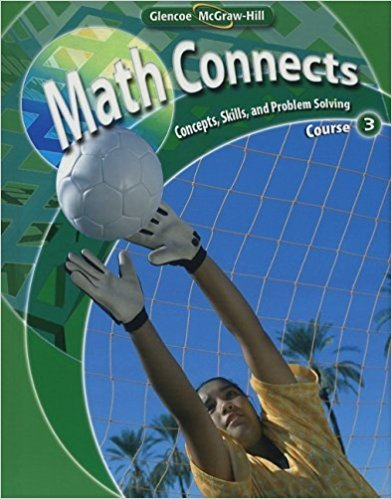×
Log in to StudySoup
Get Full Access to Math - Textbook Survival Guide
Join StudySoup for FREE
Get Full Access to Math - Textbook Survival Guide

Already have an account? Login here
×
Reset your password

Textbooks / Math / Math Connects: Concepts, Skills, and Problem Solving Course 3 0

# Math Connects: Concepts, Skills, and Problem Solving Course 3 0th Edition - Solutions by Chapter## Full solutions for Math Connects: Concepts, Skills, and Problem Solving Course 3 | 0th Edition

ISBN: 9780078740503Math Connects: Concepts, Skills, and Problem Solving Course 3 | 0th Edition - Solutions by Chapter

Solutions by Chapter
4 5 0 260 Reviews
##### ISBN: 9780078740503

Since problems from 12 chapters in Math Connects: Concepts, Skills, and Problem Solving Course 3 have been answered, more than 1653 students have viewed full step-by-step answer. Math Connects: Concepts, Skills, and Problem Solving Course 3 was written by and is associated to the ISBN: 9780078740503. This expansive textbook survival guide covers the following chapters: 12. The full step-by-step solution to problem in Math Connects: Concepts, Skills, and Problem Solving Course 3 were answered by , our top Math solution expert on 11/23/17, 04:55AM. This textbook survival guide was created for the textbook: Math Connects: Concepts, Skills, and Problem Solving Course 3, edition: 0.

Key Math Terms and definitions covered in this textbook
• Change of basis matrix M.

The old basis vectors v j are combinations L mij Wi of the new basis vectors. The coordinates of CI VI + ... + cnvn = dl wI + ... + dn Wn are related by d = M c. (For n = 2 set VI = mll WI +m21 W2, V2 = m12WI +m22w2.)

• Characteristic equation det(A - AI) = O.

The n roots are the eigenvalues of A.

• Distributive Law

A(B + C) = AB + AC. Add then multiply, or mUltiply then add.

• Dot product = Inner product x T y = XI Y 1 + ... + Xn Yn.

Complex dot product is x T Y . Perpendicular vectors have x T y = O. (AB)ij = (row i of A)T(column j of B).

• Hankel matrix H.

Constant along each antidiagonal; hij depends on i + j.

• Hermitian matrix A H = AT = A.

Complex analog a j i = aU of a symmetric matrix.

• Hessenberg matrix H.

Triangular matrix with one extra nonzero adjacent diagonal.

• Jordan form 1 = M- 1 AM.

If A has s independent eigenvectors, its "generalized" eigenvector matrix M gives 1 = diag(lt, ... , 1s). The block his Akh +Nk where Nk has 1 's on diagonall. Each block has one eigenvalue Ak and one eigenvector.

• Krylov subspace Kj(A, b).

The subspace spanned by b, Ab, ... , Aj-Ib. Numerical methods approximate A -I b by x j with residual b - Ax j in this subspace. A good basis for K j requires only multiplication by A at each step.

• Linear transformation T.

Each vector V in the input space transforms to T (v) in the output space, and linearity requires T(cv + dw) = c T(v) + d T(w). Examples: Matrix multiplication A v, differentiation and integration in function space.

• Lucas numbers

Ln = 2,J, 3, 4, ... satisfy Ln = L n- l +Ln- 2 = A1 +A~, with AI, A2 = (1 ± -/5)/2 from the Fibonacci matrix U~]' Compare Lo = 2 with Fo = O.

• Matrix multiplication AB.

The i, j entry of AB is (row i of A)·(column j of B) = L aikbkj. By columns: Column j of AB = A times column j of B. By rows: row i of A multiplies B. Columns times rows: AB = sum of (column k)(row k). All these equivalent definitions come from the rule that A B times x equals A times B x .

• Network.

A directed graph that has constants Cl, ... , Cm associated with the edges.

• Normal matrix.

If N NT = NT N, then N has orthonormal (complex) eigenvectors.

• Pivot.

The diagonal entry (first nonzero) at the time when a row is used in elimination.

• Right inverse A+.

If A has full row rank m, then A+ = AT(AAT)-l has AA+ = 1m.

• Singular matrix A.

A square matrix that has no inverse: det(A) = o.

• Solvable system Ax = b.

The right side b is in the column space of A.

• Symmetric matrix A.

The transpose is AT = A, and aU = a ji. A-I is also symmetric.

• Wavelets Wjk(t).

Stretch and shift the time axis to create Wjk(t) = woo(2j t - k).